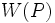# Equivalence of normality and characteristicity conditions for isomorph-free p-functor

## Statement

Let$p$ be a prime number. Suppose$W$ is a characteristic p-functor that always returns an isomorph-free subgroup of its input group. Then, the following are equivalent for a finite group$G$:

1. For every pair of$p$-Sylow subgroups$P,Q$ of$G$,$W(P) = W(Q)$.
2. For every pair of$p$-Sylow subgroups$P,Q$ of$G$,$W(P) \le Q$.
3. Each of these:
• There exists a$p$-Sylow subgroup$P$ of$G$ such that$W(P) \le O_p(G)$ where$O_p(G)$ is the p-core of$G$.
• For every$p$-Sylow subgroup$P$ of$G$,$W(P) \le O_p(G)$ where$O_p(G)$ is the$p$-core of$G$.
4. Each of these:
• There exists a$p$-Sylow subgroup$P$ of$G$ such that$W(P)$ is a characteristic subgroup of$G$.
• For every$p$-Sylow subgroup$P$ of$G$,$W(P)$ is a characteristic subgroup of$G$.
5. Each of these:
• There exists a$p$-Sylow subgroup$P$ of$G$ such that$W(P)$ is a normal subgroup of$G$.
• For every$p$-Sylow subgroup$P$ of$G$,$W(P)$ is a normal subgroup of$G$.

## Proof

Fact (1) shows that the For every versions are equivalent to the there exists versions. This proves the equivalence of the two versions of (3), the two versions of (4), and the two versions of (5). The remaining directions are each individually quite easy and are summarized below.

We prove the equivalence of (1) with (2). Within this the (2) implies (1) direction requires the use of isomorph-free. We prove the equivalence of (2) with (3). This is straightforward. We then cyclically prove the equivalence of (3), (4) and (5). Of this cyclic proof, the (3) implies (4) part uses isomorph-free via Facts (2) and (3).

From To Facts used Given data used Explanation
Condition (1) Condition (2) -- -- Immediate from the fact that$W(Q) \le Q$ on account of$W$ being a characteristic$p$-functor.
Condition (2) Condition (1)$W$ is isomorph-free If$W(P) \le Q$, then we know that$W(P), W(Q)$ are isomorphic subgroups of$Q$. By the isomorph-free condition, we must have$W(P) = W(Q)$.
Condition (2) Condition (3) -- -- If$W(P) \le Q$ for all$p$-Sylow subgroups$Q$, we have$W(P) \le \bigcap_{Q \in \operatorname{Syl}_p(G)} Q = O_p(G)$.
Condition (3) Condition (2) -- --$W(P) \le O_p(G)$ and$O_p(G) \le Q$ for all$p$-Sylow subgroups$Q$ forces$W(P) \le Q$.
Condition (3) Condition (4) Facts (2),(3)$W$ is isomorph-free We have$W(P) \le O_p(G) \le P \le G$. By Fact (2),$W(P)$ is characteristic in$O_p(G)$. Separately,$O_p(G)$ is characteristic in$G$. Fact (3) now gives that$W(P)$ is characteristic in$G$.
Condition (4) Condition (5) Fact (4) -- Fact-direct
Condition (5) Condition (3) Fact (5) By definition of p-core,$W(P)$ being normal forces$W(P) \le O_p(G)$.